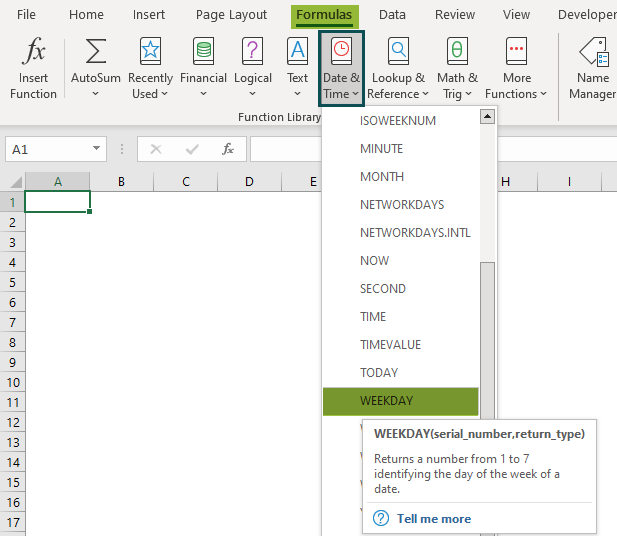# WEEKDAY Function In ExcelArticle byExcelMojo Team## What Is WEEKDAY Function In Excel?

The WEEKDAY function in Excel matches the weekday with the date, in the form of an integer, from 1-7 i.e., 1[Sunday] to 7[Saturday], representing the days of the week. The WEEKDAY function in Excel is an inbuilt function so we can insert it from the “Function Library” or directly enter it as a formula.

For example, we will apply the WEEKDAY in Excel to get the weekday on the date in cell A1.

Select cell B2, enter the formula =WEEKDAY(A2, and press the “Enter” key.

The output is ‘4’, as shown above. Therefore, the day on the date is “Wednesday”.

Special Note: The output of the the WEEKDAY formula in Excel when the “Return type” differs are shown in the table below.

###### Key Takeaways
• The WEEKDAY function in Excel is from the “Date & Time” category in the “Function Library”, which helps us find the weekday for a given day in a dataset.
• We can use conditional functions such as IF() and CHOOSE() with the Weekday() function to get an alternate output other than the weekday integer value.
• The #NUM! error occurs,
• When the “return_type” is out of range from the “return_type” range.
• When the “serial_number” is out of range from the current date.

### WEEKDAY() Excel Formula

The Syntax of the WEEKDAY Excel formula is,

The arguments of the WEEKDAY Excel formula are,

• serial_number: It is the mandatory argument. It is a sequential number representing the date of the day we are trying to find.
• return_type: It is the optional argument. It specifies which integers are to be assigned to each weekday.

### How To Use WEEKDAY Excel Function?

We can use the WEEKDAY Excel Function in 2 methods, namely,

1. Access from the Excel ribbon.
2. Enter the worksheet manually.

#Method 1 – Access from the Excel ribbon

Choose an empty cell for the output >select the “Formulas” tab > go to the “Function Library” group > click the “Date & Time” drop-down > select the “WEEKDAY” function, as shown below.

The “Function Arguments” window appears. Enter the values for the arguments in the “serial_number” and “return_type” fields > click “OK”, as shown below.

#Method 2 – Enter the worksheet manually

• Select an empty cell for the output.
• Type =WEEKDAY() in the selected cell. [Alternatively, type “=W” and double-click the WEEKDAY function from the list of suggestions shown by Excel.]
• Enter the arguments as cell values or cell references in excel.
• Press the “Enter” key.

#Basic Example

We will find the weekdays using WEEKDAY function in Excel.

In the table, the data is,

• Column A contains the Date.
• Column B contains the Output.

The steps to find the weekday using WEEKDAY function in Excel are as follows:

1. First, select cell B2 and enter the formula =WEEKDAY(A2).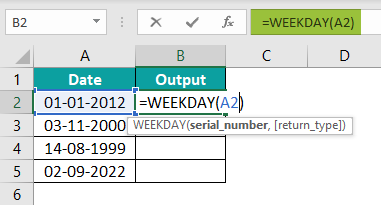2. Press the “Enter” key. The output is ‘1’, as shown below.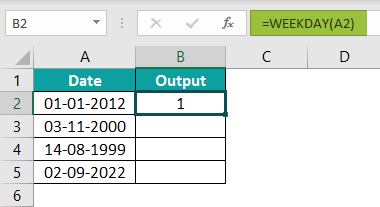3. Drag the formula from cell B2 to cell B5 using the fill handle.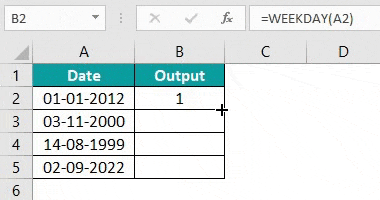The output is shown above. Column C, weekday names, are for our reference.

### Examples

Let us understand using WEEKDAY in Excel with some advanced scenarios.

#### Example#1

We will find the weekdays using the IF() excel function and the WEEKDAY function in Excel.

In the table, the data is,

• Column A contains the Date.
• Column B contains the Output.

The steps to find the weekdays using the WEEKDAY function in Excel and the IF() functions are:

Step 1: Select cell B2, enter the conditional IF() formula =IF(WEEKDAY(A2)=1,”Sunday”,IF(WEEKDAY(A2)=2,”Monday”,IF(WEEKDAY(A2)=3,”Tuesday”,IF(WEEKDAY(A2)=4,”Wednesday”,IF(WEEKDAY(A2)=5,”Thursday”,IF(WEEKDAY(A2)=6,”Friday”,IF(WEEKDAY(A2)=7,”Saturday”)))))))).

Step 2: Press the “Enter” key. The result is shown below.

Step 3: Drag the formula from cell B2 to B8 using the fill handle. The output is shown below.

#### Example#2

We will find the working days and weekends using the IF() and the WEEKDAY function in Excel.

In the table, the data is,

• Column A contains the Date.
• Column B contains the Output.

The steps to find the weekdays and working days using WEEKDAY() and the IF() functions are:

Step 1: Select cell B2, enter the formula =IF(WEEKDAY(A2,2)<6,”Working Day”,”Weekend”).

Step 2: Press the “Enter” key. The result is shown below.

Step 3: Drag the formula from cell B3 to B8 using the fill handle. The output is shown below.

#### Example #3

We will find the weekdays using the CHOOSE() function and the WEEKDAY function in Excel.

In the table, the data is,

• Column A contains the Date.
• Column B contains the Output.

The steps to find the weekday using WEEKDAY formula in Excel and the CHOOSE() function are:

Step 1: Select cell B2 and enter the formula

=CHOOSE(WEEKDAY(A2),”Monday”,”Tuesday”,”Wednesday”,”Thursday”,”Friday”,”Saturday”,”Sunday”).

Step 2: Press the “Enter” key. The result is shown below.

Step 3: Drag the formula from cell B2 to B13 using the fill handle. The output is shown below.

### Important Things To Note

• In the WEEKDAY function in Excel, the “return_type” argument takes the default value as 1.
• The “serial_number” argument should be a cell reference containing a date or a date returned from another function or formula.
• When we give any input other than the 1 to 7 integer values, for the “return_type” argument, then the weekdays are repeated. [For example, if we enter 8, we will get Sunday as a return value, as after 7 days of the week, once again, it’s a Sunday.]

How does the WEEKDAY function work in Excel?

The WEEKDAY function works in Excel as follows:

1. Select an empty cell to enter the Formula.
2. Type =WEEKDAY() in the selected cell. Alternatively, type “=W” and double-click the WEEKDAY function from the list of suggestions shown by Excel.
3. Press the “Enter” key.

Let us understand with the following example.

We have the date values in Column A. Select cell B2, enter the formula =WEEKDAY(A2), and press the “Enter” key. Once we get the result, drag the formula to cell B3.

We get the output as ‘4’ and ‘2’, which means the day on the dates are “Wednesday & Monday”.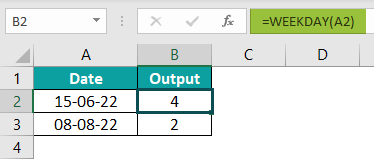WEEKDAY function in Excel definition?

The WEEKDAY function in Excel calculates and returns the value representing the day of a given date. The output returned can take any value from 0 to 7. The user can specify the day from which this value is calculated, i.e., it can start from 0 or 1. The function works with the dates entered as a result of some other function, the dates stored as text or the dates entered as text.

The formula of the WEEKDAY in Excel is =WEEKDAY(serial_number,[return_type])

Where is the WEEKDAY function in Excel?

We can insert the WEEKDAY function in Excel using the following steps:

First, choose an empty cell for the output > select the “Formulas” tab > go to the “Function Library” group > click the “Date & Time” drop-down > select the “WEEKDAY” function, as shown below.